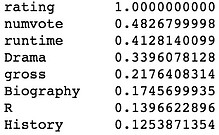top of page#### Problem statement

Luther is the latest prediction engine developed by Prophet42, Inc. to help customers in the movie industry. It aims to predict the gross and rating of a movie before it hits the theaters based on information from similar movies.

#### Strategy

1. Build a movie database with web scraping technology (Python BeautifulSoup).

2. For each target variable (rating for example), find the most relevant feature variables, and build a model for prediction.

3. Test the efficacy of the model using train/test split sets and cross validation technique.

#### Execution

We accessed the web pages of 10,000 movies from imdb.com and saved each page to a local html document. From each html file, information such as title, revenue(gross), budget, rating, and other relevant information was extracted using BeautifulSoup and regex. They were collectively saved to a pandas dataframe.After removing movies with incomplete information, we ended up with a pandas dataframe of 3902 rows/movies/observations. We then added a series of "dummy variable" columns based on genre and MPAA rating. The elements inside those columns were given a value 0 or 1 based on whether the movie has the corresponding attribute. For example, an "R" rated movie of "Drama" genre has "R" column value of 1, "Drama" column value of 1, "PG" column value of 0, and "Comedy" column value of 0.The correlation matrix provides the most relevant features for a given target variable ("rating" in this case).Note that though "numvote" (number of votes on imdb) has the highest correlation with the target variable, we cannot use it for our model since its value is unavailable before a movie hits the theaters.

The final candidates for "rating" model are: 'numvote', 'runtime', 'Drama', 'gross', 'Biography', 'R', 'History', 'War', 'PG', 'Comedy', 'Horror'

As the first attempt, a standard linear regression model was used.The following workflow was applied to find a better model.

step 1. standard linear regression

step 2. linear regression with Lasso and determine the best alpha

reg_lassocv = LassoCV(alphas= [1e-8, 1e-7, 1e-6, 1e-5, 1e-4, 1e-3, 1e-2, 1e-1, 1, 1e2, 1e3])

step 3. with the best alpha from step 2, use PipeLine to create polynomial feature fit with Lasso

est_poly = make_pipeline(PolynomialFeatures(2), Lasso(alpha = 1e-3))

step 4. analyze coefficients from step 3 and create a statsmodels formula using items with non-zero coefficients

lm1 = smf.ols(model_formula , data=df_rating)

fit1 = lm1.fit_regularized(L1_wt = 1, alpha=1e-3)

step 5. simplify the model by removing items with high p-values in smf.ols fit

step 6. cross validation using KFold from sklearn

for train_index, test_index in kf.split(df_rating):

df_train = df_rating.iloc[train_index, :]
df_test = df_rating.iloc[test_index, :]

A better model is a 2nd degree polynomial fit using statsmodel with an alpha = 0.001 for Lasso.The results for KFold validation (n = 5) are:

• 0.211041628636

• 0.238587980349

• 0.315476940281

• 0.294975747774

• 0.233990161157

Using similar technique, another model was optimized for "gross" target variable.#### Summary

This project made substantial use of the following skills:

web scraping, pandas data cleaning, feature selection, model selection, linear regression, cross validation

The models' performance could have been better if some omitted features were added to the model, however, the core of the project remains unchanged. Such omissions stay true to the original purpose/problem statement: to predict rating/gross using data before a movie hits the theaters.

bottom of page